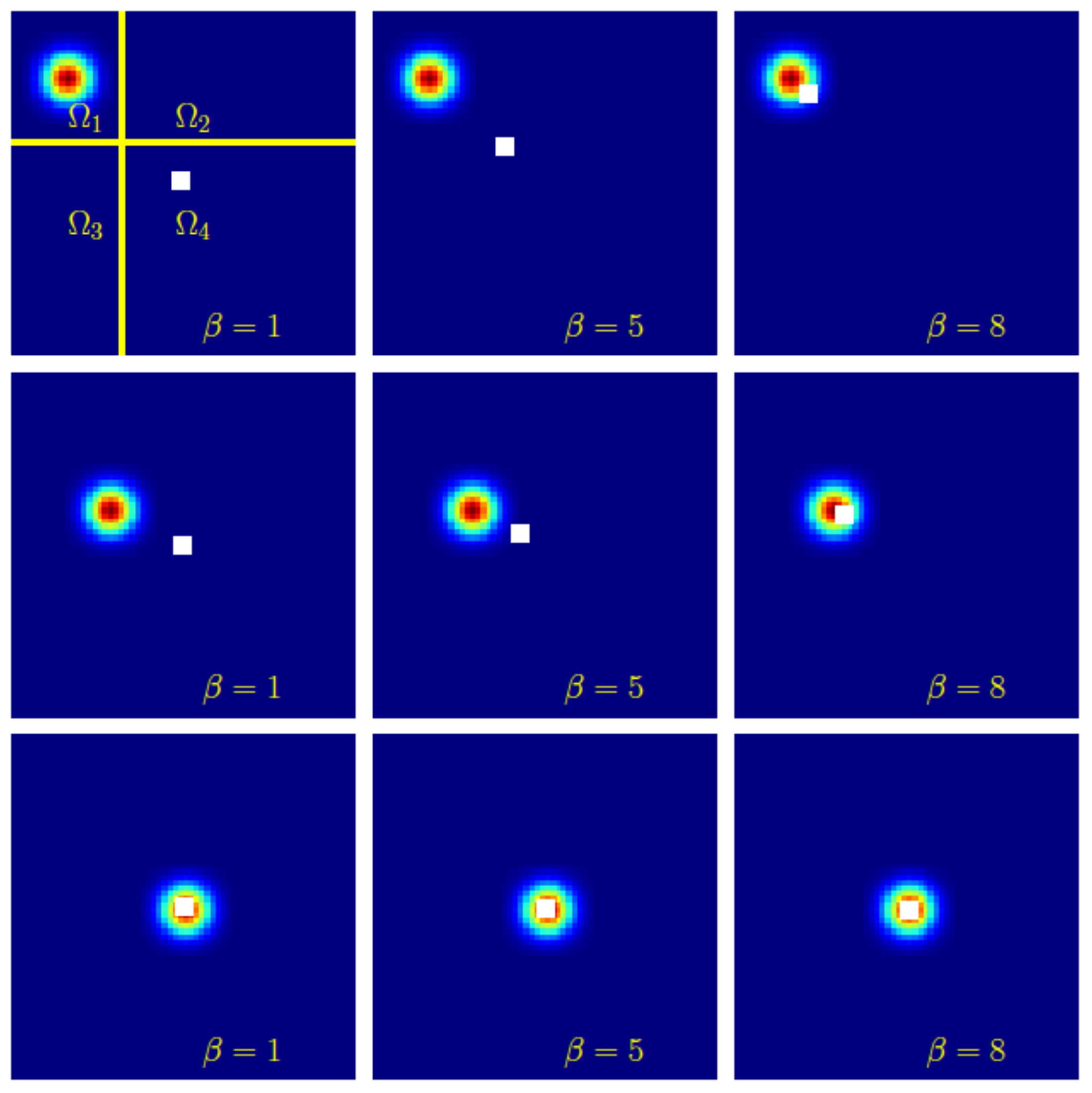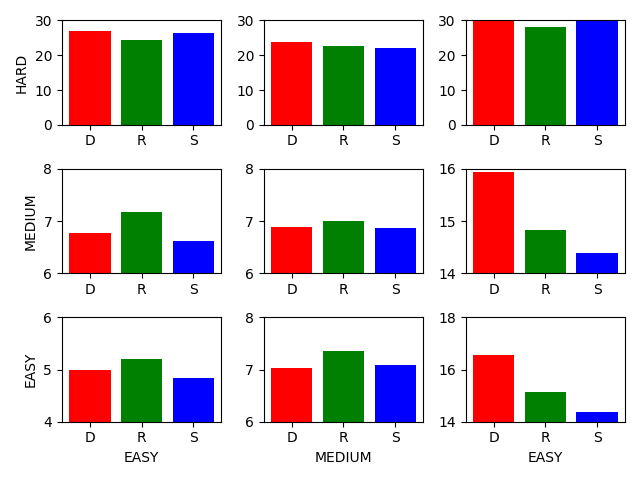Removing the Bias of Integral Pose Regression

Kerui Gu1, Linlin Yang1,2, Angela Yao1
1National University of Singapore, 1University of Bonn

Abstract

Heatmap-based detection methods are dominant for 2D human pose estimation even though regression is more intuitive. The introduction of the integral regression method, which, architecture-wise uses an implicit heatmap, brings the two approaches even closer together. This begs the question -- does detection really outperform regression? In this paper, we investigate the difference in supervision between the heatmap-based detection and integral regression, as this is the key remaining difference between the two approaches. In the process, we discover an underlying bias behind integral pose regression that arises from taking the expectation after the softmax function. To counter the bias, we present a compensation method which we find to improve integral regression accuracy on all 2D pose estimation benchmarks. We further propose a simple combined detection and bias-compensated regression method that considerably outperforms state-of-the-art baselines with few added components.

Bias of Integral Pose RegressionLeft image illustrates the bias of integral pose regression in 1D. The distribution is a symmetric Gaussian plus a tail with value of 0s. Before normalization, the argmax (detection) is same with expected value (integral pose regression). After normalization using softmax function, the value of the tail becomes positive (considering exp(0)=1), the expected value is biased to the right side.
Right figure presents the different distributions of normalized heatmaps plus a white square (expected value) under different softening parameter beta. We can see that only when the activated area appears at center of the image will bias disappear. Large beta relieves the problem to some extent, but it causes backpropagation problem.

Detection Versus Integral RegressionLeft: Further division of MSCOCO benchmark. In these sub-divisions, few number of joints present, heavy occlussion cases consist of visually hard samples.
Middle: Comparison of training speed between detection and regression. Detection trains much faster than regression especially in the initial epochs.
Right: Comparions of performance on the nine divided sub-benchmarks. Regression preformances better in visually hard samples.

Results on BenchmarksEmpirical results on human pose dataset MSCOCO and MPII, hand pose dataset RHD.

Bibtex

@inproceedings{gu2021removing,
title={Removing the Bias of Integral Pose Regression},
author={Gu, Kerui and Yang, Linlin and Yao, Angela},
booktitle={Proceedings of the IEEE/CVF International Conference on Computer Vision},
pages={11067--11076},
year={2021}
}